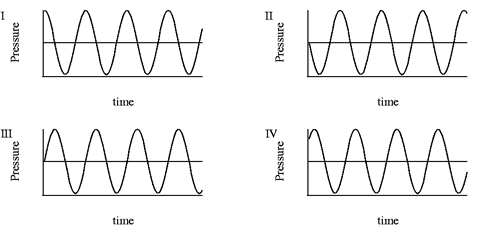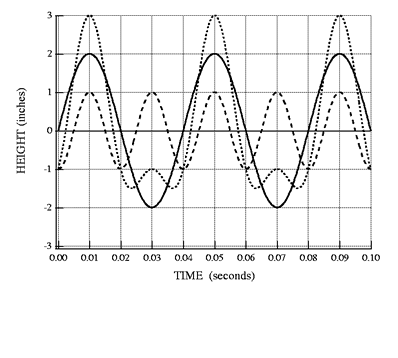Back to "Answer-This" Listing "Answer This" Questions Chapter 6 6-A. Phase. Which pair, if any, of the waves in the figure below are in phase? Which pair, if any, are 180o out of phase?Answer: Being in phase implies that the cycles in the two waves occur in unison: crests occur at the same time, troughs occur at the same time, etc. No pair of these fit that description. (Note that I and IV are only a small amount out of phase.) Being 180o out of phase means that the crest of one wave occurs when the trough of the other does and vice versa. Waves II and III have this property. ____________________________________ 6-C. Graphical sum of two sinusoidal waves. Figure 6-6 of Fundamentals of Sound shows displacement versus time curves for two sinusoidal waves for motion at a particular position on a string. Plot the complex wave formed by the sum (or superposition) of the two sinusoidal waves. Answer: The two sinusoidal curves are shown as solid and dashed curves. The sum of the two is shown as the dotted curve. Note, for example, that this curve reaches a height of 3 in at t = 0.01 s, which is the sum 1 in + 2 in, the values of the sine curves. At t = 0.03 s, it has height = -1 in = (1 – 2) in, because the sine curves are at 1 in and -2 in. The total curve is definitely not sinusoidal.6-F. Repeat time of a complex wave. Three complex sound waves are created by adding combinations of frequencies in the harmonic series { f0 = 10 Hz, 2f0 = 20 Hz, 3 f0 = 30 Hz, 4 f0 = 40 Hz, etc.}. The constituents of the three waves are as follows: Wave 1 contains first and second harmonics. Wave 2 contains first, second, and fourth harmonics. Wave 3 contains first, fifth, and tenth harmonics. Which of the following is true? a) The repeat time of all three waves is the same. b) The repeat time of all three waves is 1/10 s. c) All three waves may have different repeat times. d) The repeat time of the three waves depends on the phases of the constituent frequencies, so we can’t tell anything about the repeat times. e) Both A and B.                    f) Both C and D.                         g) None of the above.   Answer: The correct answer is e). All three waves contain the fundamental. Thus after the period of the fundamental, all the harmonics in all three waves will have repeated an integral number of times, once for the fundamental, two times for the second harnomic, etc. This is the shortest time that all the components of every wave will be back to their starting shapes, so T0 = 1/f0 is the repeat time in all three cases. In each case it is 1/10 s. Back to "Answer-This" Listing     © William J. Mullin